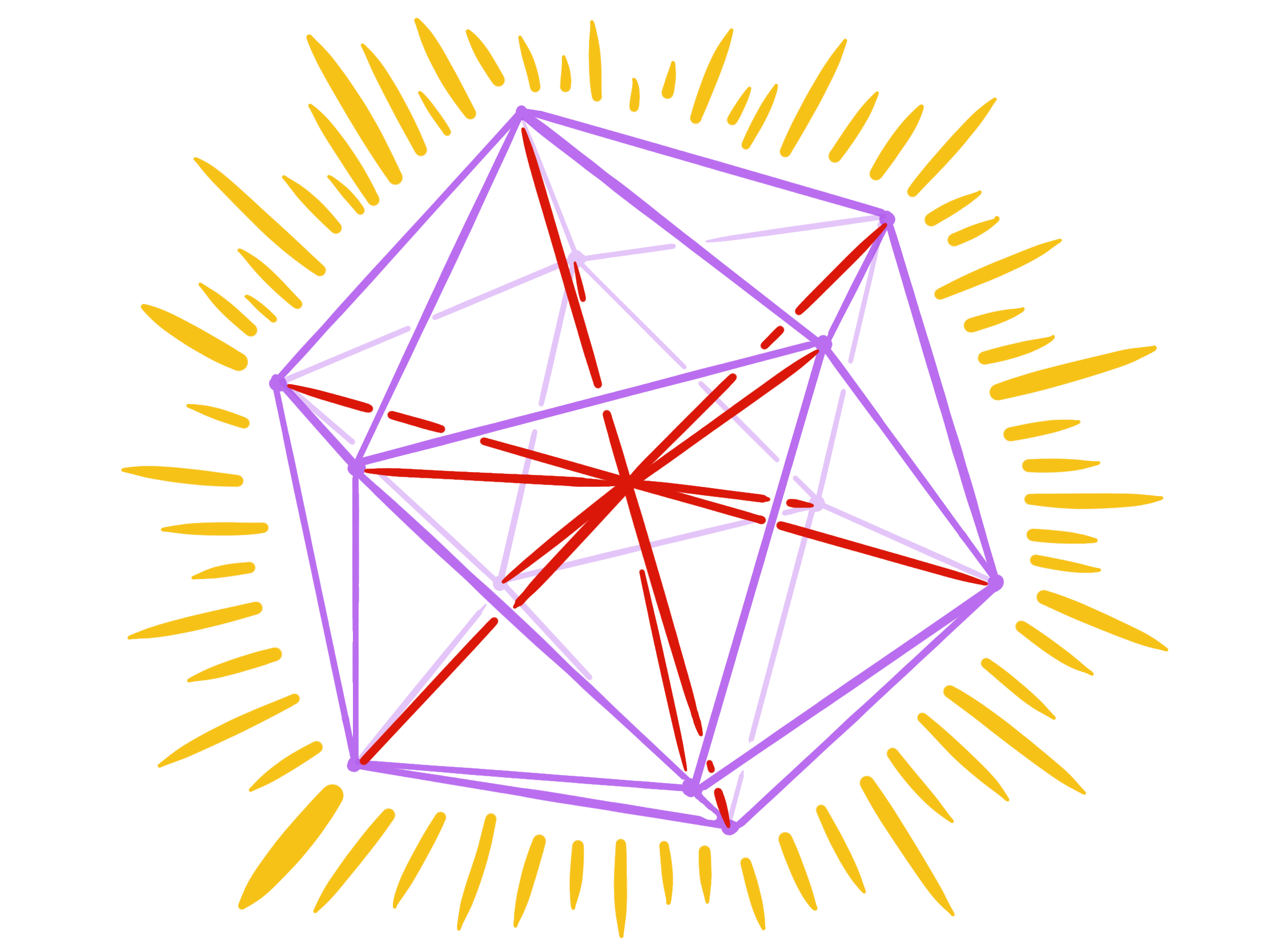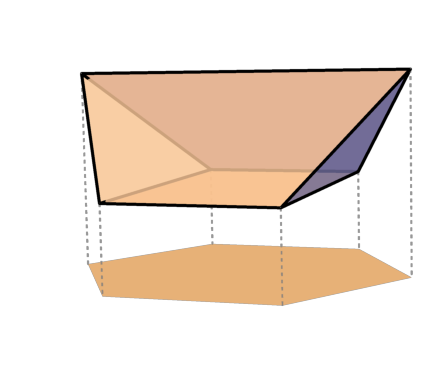# Research

My papers are also listed on arXiv, MathSciNet, Google Scholar, and my CV

## Research Papers

The Green-Tao theorem and a relative Szemerédi theoremGraph regularity method and applicationsArithmetic regularity and applications

Cayley graphs

Equiangular lines and spectral graph theoryIncidence geometryNonnegative rank and extension complexityIndependent sets and graph homomorphismsSphere packing and energy minimization

Large deviations in random graphs

Graph limits

Random matrices

Directed graphs and tournamentsExtremal and Ramsey graph theory

More sums than differences sets

Other topics

## Expositions and surveys

Older expository papers and notes